• 半波偶极子天线是一种结构简单的基本线天线，也是一种经典的、迄今为止使用最广泛的天线之一。偶极子天线是研究天线的基础，最常用的就是半波偶极子天线，其具有很多特性，比如辐射特性阻抗特性，波长缩短效应，谐振...
• 偶极子天线是射频识别中结构简单，易于制作，应用广泛的天线之一。本文对半波偶极子天线的回波损耗s11、阻抗及方向性进行简单的研究，进而在半波偶极子天线的基础上，设计出弯折个数n为3的弯折偶极子天线，并且要求...
• 平面偶极子天线具有重量轻、体积小、成本低、便于集成和组成阵列等优点被广泛应用于各种移动通信的天线中。这类天线应用Balun结构馈电方式，不但克服了平面天线带宽窄的缺点，在相对带宽40％的频带内，实现了天线的...
• 本文设计了一种双面印刷偶极子天线，采用双层平面偶极子结构降低了交叉极化电平，利用平衡巴伦匹配获得双谐振点拓展带宽。最后达到了40%的回波损耗小于-10dB的阻抗带宽，带内增益5-9dBi，交叉极化电平小于-50dB的...
• 为了拓宽偶极子天线的工作带宽，实现天 线 的 小 型 化，设 计 了 一 种 ＶＨＦ／ＵＨＦ波段的偶极子天线结构，融 合 分 布.式加载匹配技术，并借助矩量法和遗传算法对天线的辐射性能进行优化。模拟与实验测试结果...
• 一、印刷偶极子天线 1、设计指标 中心频率：2.45GHz 相对介电常数：4.4 介质板厚度：1.6mm 2、天线结构 如图所示为设计的微带巴伦馈线印刷偶极子天线的结构模型，该天线属于半波偶极子天线的变形。整个天线结构大致...
一、印刷偶极子天线
1、设计指标
中心频率：2.45GHz相对介电常数：4.4介质板厚度：1.6mm
2、天线结构
如图所示为设计的微带巴伦馈线印刷偶极子天线的结构模型，该天线属于半波偶极子天线的变形。整个天线结构大致可以分为5部分，即介质层、偶极子天线臂、微带巴伦线、微带传输线和天线馈电面。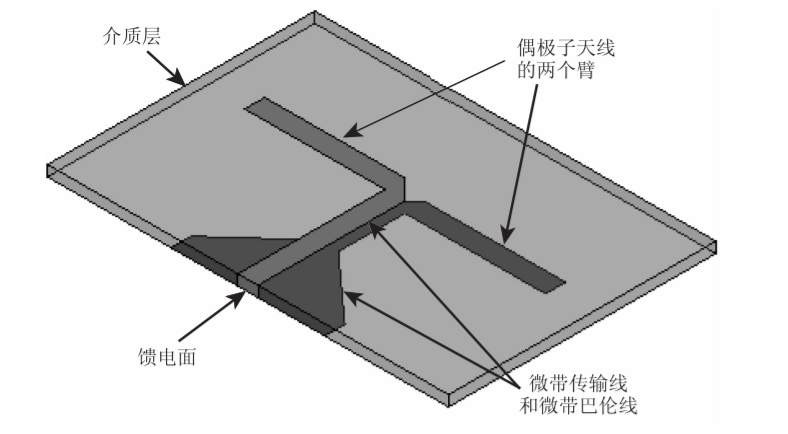介质层的材质为环氧树脂玻璃纤维板( FR4)，其相对介电常数

ε

r

\varepsilon_{r}

=4.4。在介质层的两面分别敷有良导体的金属传输线，构成偶极子天线的两个臂、微带传输线和微带巴伦线。激励信号从天线馈电点处馈入，经过微带巴伦结构和微带传输线传输到偶极子天线的两个臂。在微带传输线上，电流方向相反，因此不会辐射电磁波。在偶极子天线的两个臂上，金属片的电流方向相同，因此会辐射电磁波。由半波偶极子天线的理论分析可知，天线两个臂的总长度约为1/2个工作波长。 我们知道偶极子天线是一个对称结构，传输线上的馈电电流必须是对称分布的。若是馈线采用双传输线结构，因为双传输线的电流为对称分布，所以天线的电流亦为对称分布。然而，若是馈线采用同轴线结构，因为同轴线内外导体并不对称，所以天线上的电流也不会对称分布，从而会影响天线的性能。为了保证偶极子天线上电流的平衡，通常在天线和同轴线之间插入一个不平衡到平衡的转换器，即微波巴伦，它可以将不平衡的电流转换成平衡的电流。上图中的三角形结构就是一一个简单的微波巴伦，它可以实现不平衡到平衡的转换。 我们知道半波偶极子天线的输人阻抗约为73.2

Ω

\Omega

,而馈电端口同轴线的特性阻抗一般是 50

Ω

\Omega

，若将同轴线直接连接至半波偶极子天线上，就会有阻抗不匹配的问题。三角形微带巴伦和微带传输线一起起到阻抗转换的作用，其作用相当于1/4波长阻抗转换器，可以调节传输线的长度和三角形的大小，还可以调整馈电面的输入阻抗。
二、模型创建
1、设计参量
设计天线的中心频率为2. 45GHz,若在自由空间中传播，对应的工作波长约为波长约为122mm。若在全部填充以FR4材质的介质中传播，其对应的工作波长约为58mm。若我们采用自由空间波长，则半波偶极子的长度约为61mm。若我们采用介质中的波长，则半波偶极子的长度约为29mm。因为印刷偶极子天线同时包含介质与自由空间，所以实际的半波偶极子的长度应该介于29mm和61mm之间，我们取二者的平均值45mm作为半波偶极子长度的初始值，然后再使用HFSS软件分析出半波偶极子长度的实际值。在相对介电常数为4.4、厚度为1. 6mm的FR4介质板上，微带线结构对应的导波波长约68mm，1/4波长约为17mm。对于设计中的微带传输线，长度应该略大于17mm，设计中初始值取22mm。 对于三角形的微带巴伦结构，两个直角边的长度初始值分别取12mm和10mm。设计中，金属片的宽带初始值都取3mm。
变量意义变量名变量值(单位: mm)介质层厚度H1.6传输线宽度W13传输线长度L122偶极子金属片宽度W23偶极子一个臂长度L221巴伦三角形侧边直角边长L310巴伦三角形底边直角边长L412微波巴伦长方形部分的宽度W33
参数化模型：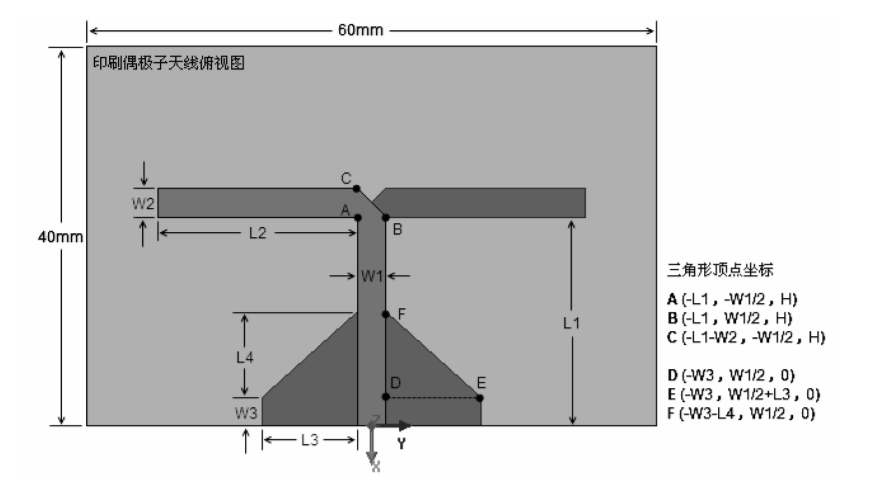2、HFSS中的参量设定
进入设计属性（design properties）将上述参量添加进去，如下图所示：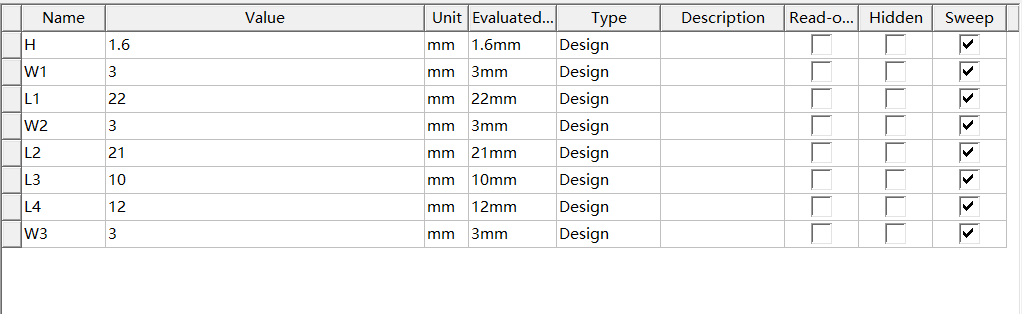3、模型建立
所建立模型如下，在模型建立的过程中使用了镜像复制（Mirror Duplicate）、镜像（Mirror）、合并（Unite）、平移（Move）等来提高效率。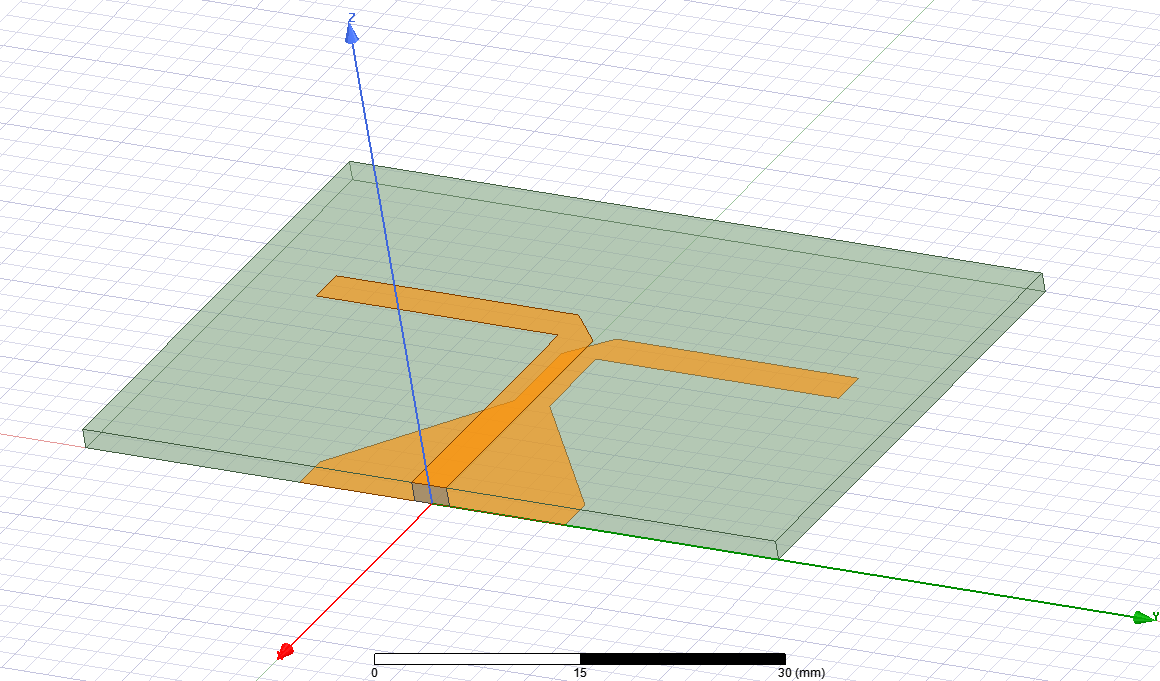激励端口设置如下，使介质板上下的微带线相连。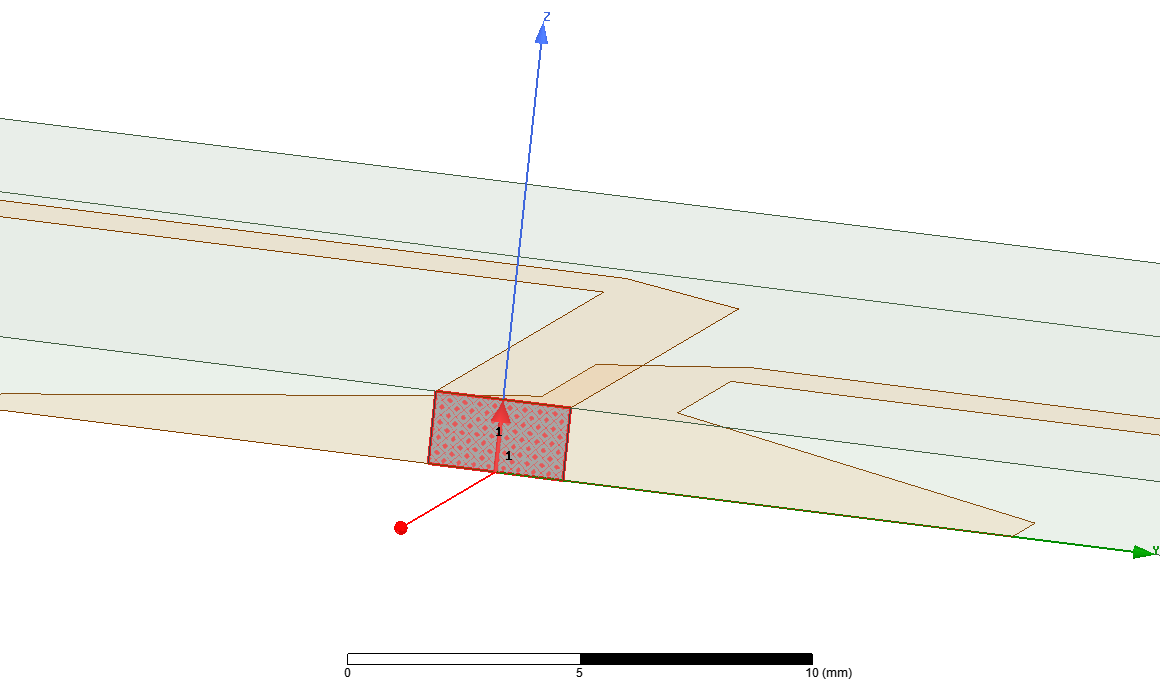三、仿真结果
1、回波损耗
S(1,1)结果如下，从分析结果可以看出，设计的偶极子天线的中心频率约为2.45GHz，

S

11

S_{11}

< -10dB的相对带宽BW =(2. 73 -2.25)/2. 45=19.6%。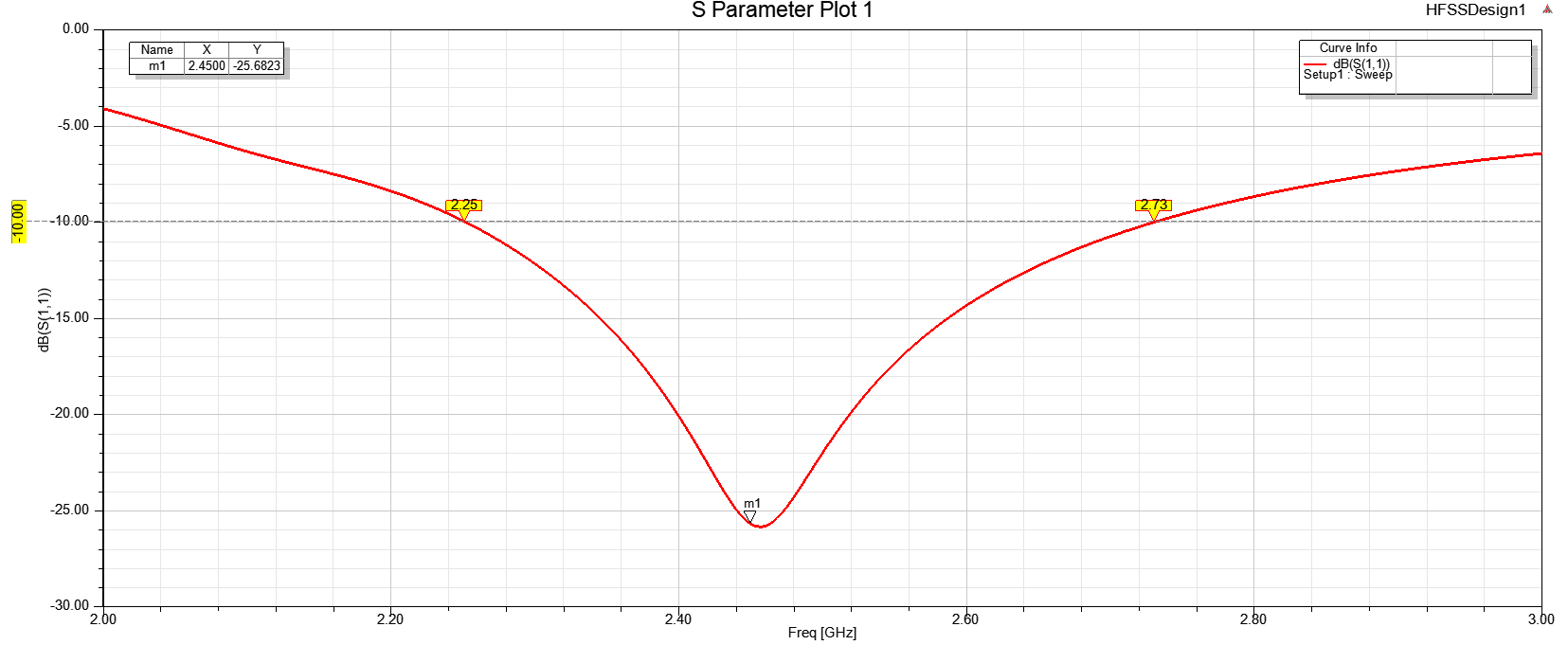2、输入阻抗
查看输入阻抗的设置如下：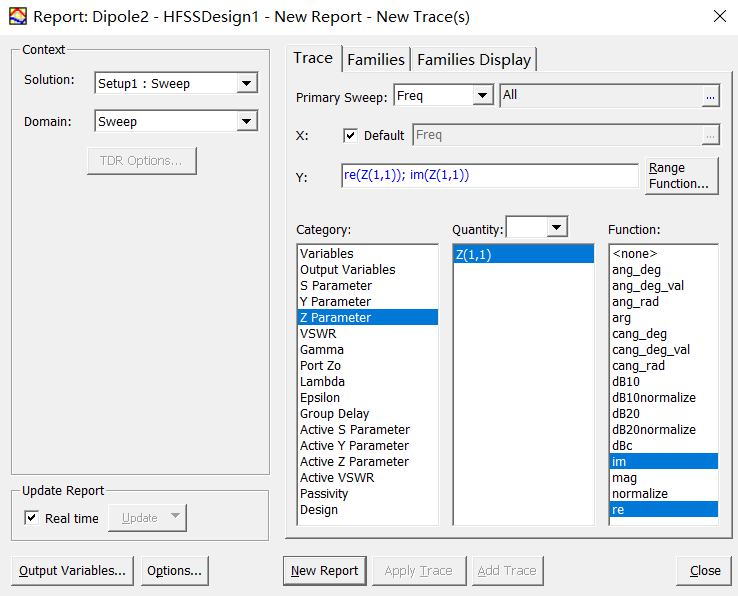点击【New Report】生成如下结果：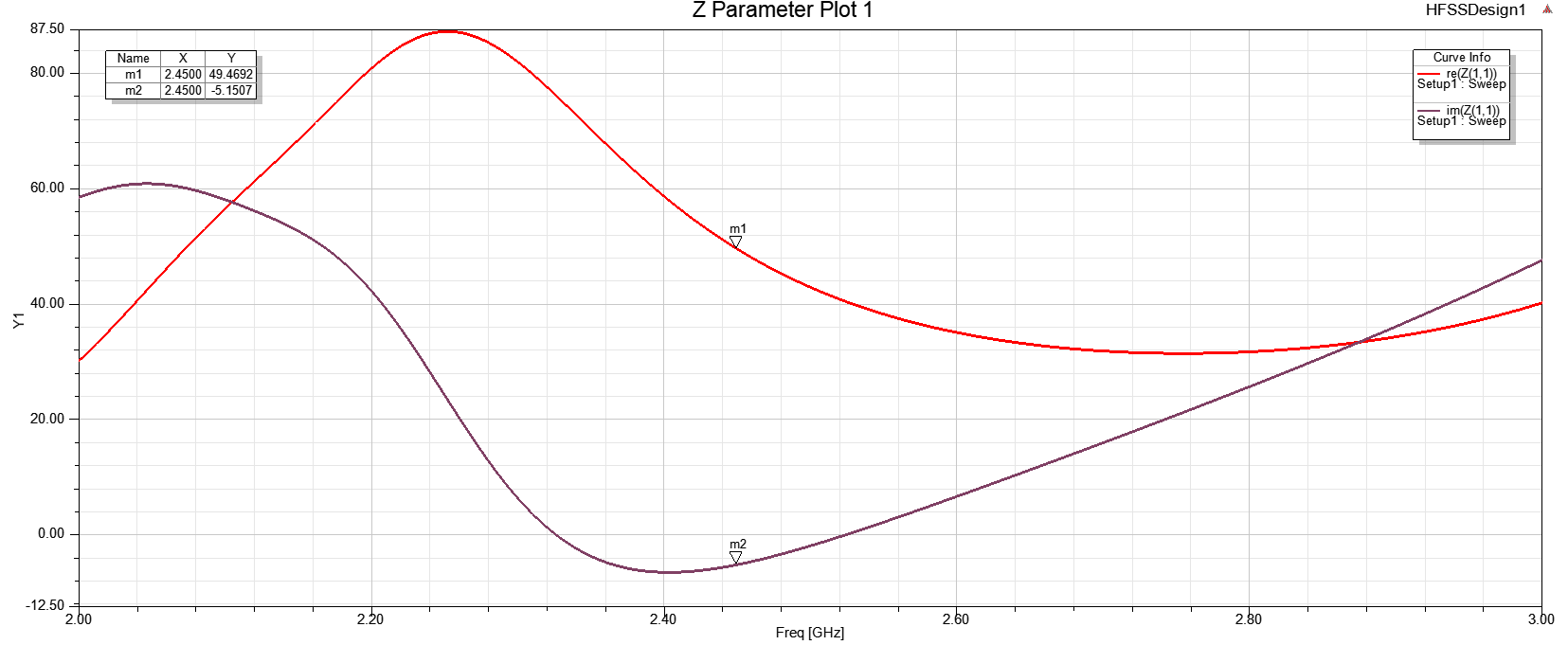从结果可以看出，在中心频率2.45GHz处，天线的输入阻抗为（49.46-j5.15）

Ω

\Omega

。可见初试的天线结构已经达到了良好的阻抗匹配。
3、方向图
天线方向图是在远场区确定的，要查看天线远区场的计算结果，首先需要定义辐射表面。定义辐射边界为无限大空间（Infinite Sphere），设置角度界面如下：下面我们给出设计的印刷偶极子天线的三维立体增益方向图：四、分析天线臂的长度对中心频率的影响
虽然当前设计的天线的初始结构已经达到了性能要求，但多数时候天线设计是不可能一步到位的，这就需要我们根据所学的理论知识，调整天线的结构参数，然后再交由HFSS仿真分析出能够达到设计要求的结构参数值。这里我们首先调整天线臂的长度，借助于HFSS的参数扫描分析功能分析出其对天线中心频率的影响。添加参数扫描分析项如下，因为设计中L2表示天线臂的长度，此处将L2进行扫描分析。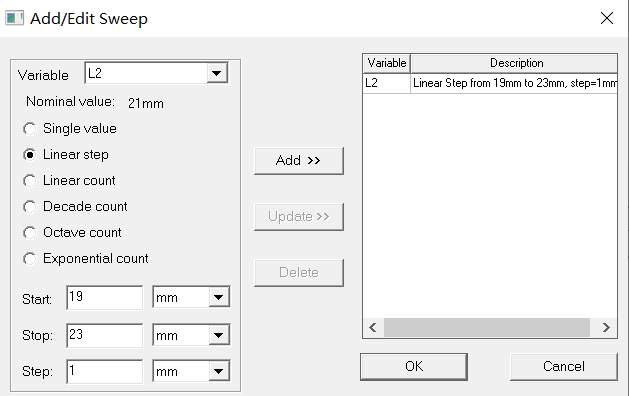分析结果如下：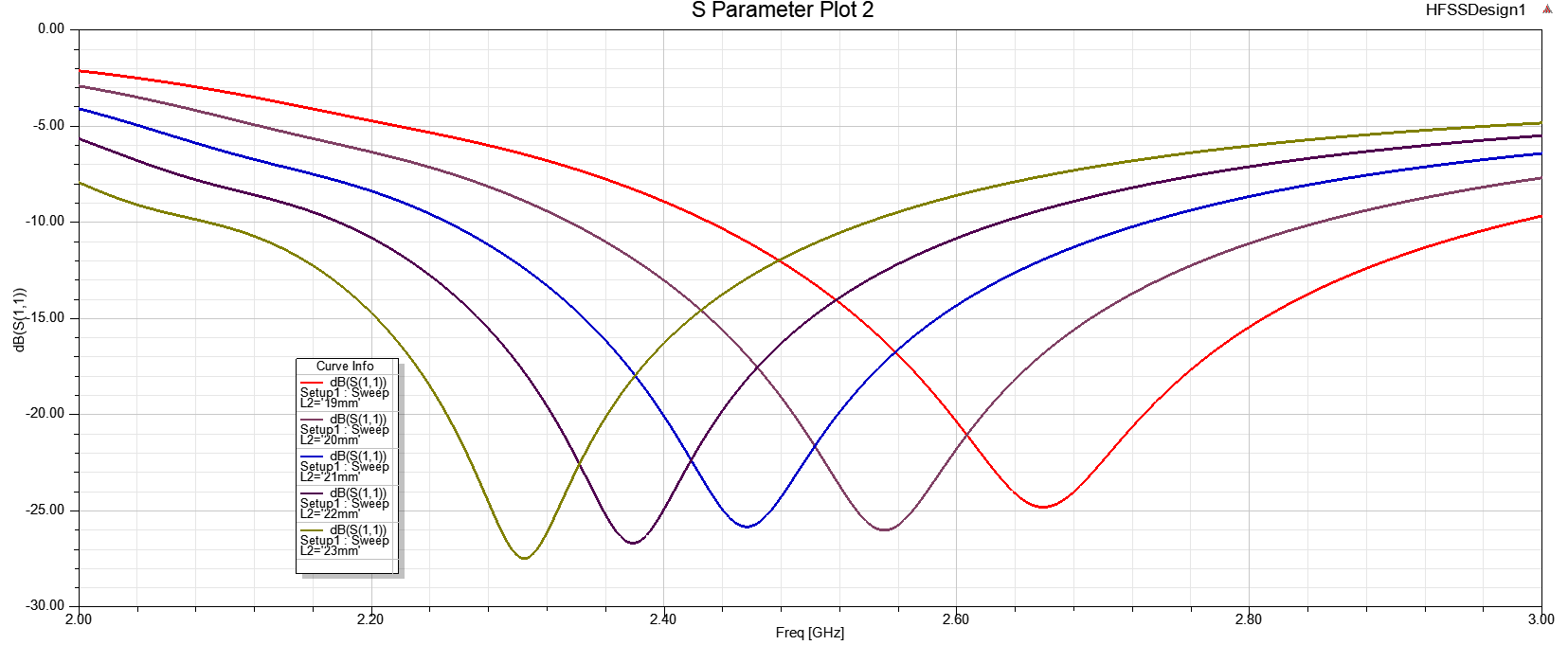从结果可以看出，天线的谐振频率随着天线比的增大而减小。相同的方法对L3扫描，可得出平衡三角形的大小对带宽的影响如下：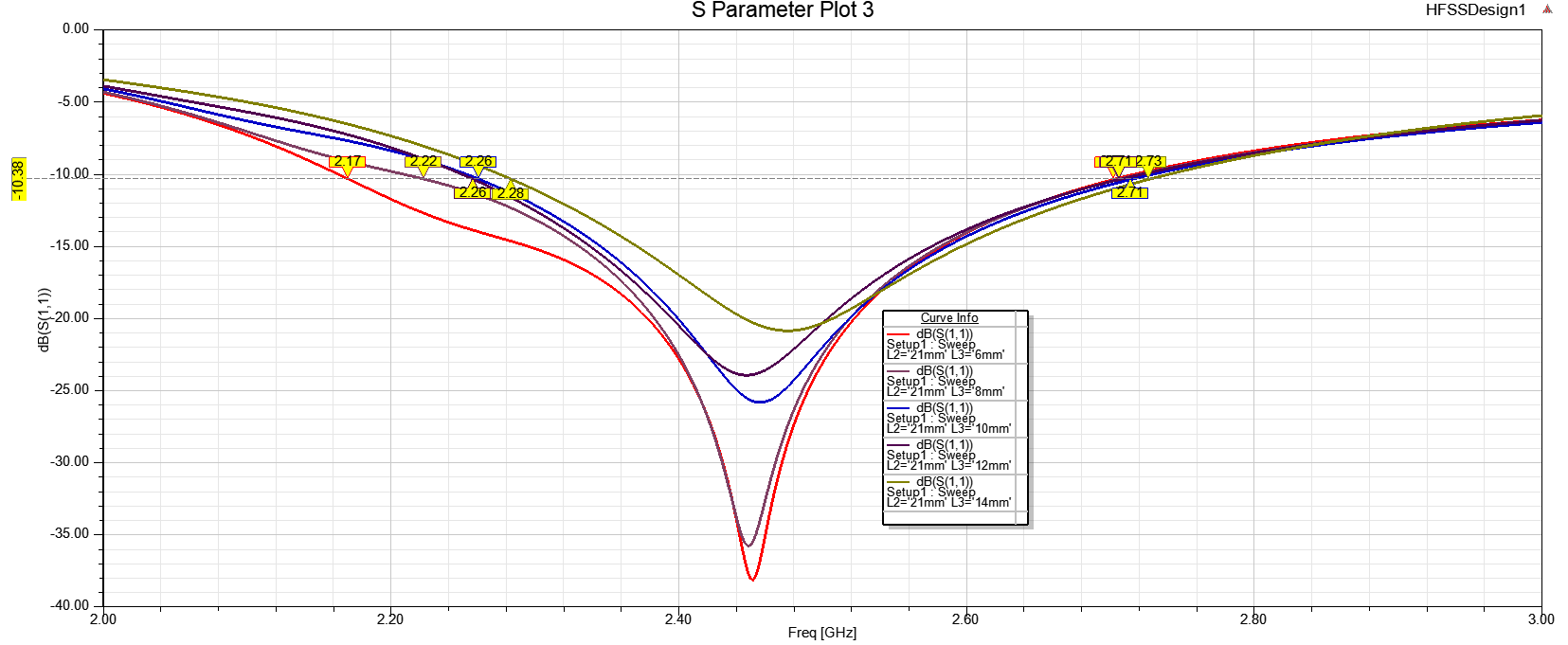可以看出平衡三角形的直角边长L3增大，天线的带宽随之减小。
展开全文射频工程
• 一种新型宽带低交叉极化印制偶极子天线，周占伟，杨仕文，设计出一种新型宽带低交叉极化印制偶极子天线，该天线采用双层平面Balun偶极子结构，减小天线横向交叉极化的电场分量，使天线不仅�
• 介绍了一种运用于无线局域网的小型化印刷偶极子天线，它采用对偶极子两个臂开槽结构，使其获得双频带和小型化的特性。采用基于耦合馈电的巴伦馈电方式，使天线具有宽带匹配、结构简单、制作方便、易于集成到无线通信...
• 基于传统的偶极振子天线的原理和结构特点,结合微带天线的优势,改进设计了一种中心工作频率为2.45GHz的微带贴片偶极子天线,缩小了天线尺寸。设计过程中使用Ansoft HFSS软件搭建天线的模型并进行仿真。制作的天线利用...
• 本文介绍了一种可用于WLAN的印刷偶极子天线，采用巴伦来耦合馈电40nnn×50mm的尺寸，结构非常简单，覆盖了WLAN的两个频段(2.4GHz和5．8GHz)，适用于WLAN系统。
• 摘 要：研究了应用时域有限差分法(FDTD)方法分析了平面Balun偶极子天线。结果表明，FDTD方法分析平面结构的天线非常有效。通过计算，可以得到天线的回波损耗和辐射特性，同时与实验结果相比，具有较好的一致性，...微带天线
• 为了快速地设计一款应用于无线局域网络(WLAN)的双面结构的具有双频段、宽频带、小型化特性的偶极子天线,将偶极子贴片分别放置于介质基板的两侧,并采用微带巴伦线馈电的方式,以实现更好的宽带匹配。在偶极子的两臂...
• 为了扩展天线的阻抗带宽和改善天线的方向性，提出一种采用了反射结构的新型印制偶极子天线。分析微带巴伦和印制偶极子的工作原理，延伸并成形天线的地平面以得到反射结构。采用V形地平面反射天线向馈电结构的辐射，...
• 行业分类-电子电器-一种太赫兹片上集成偶极子天线过渡结构.zip
• 针对周期复合左／右手（ Ｃｏｍｐｏｓｉｔｅ　Ｒｉｇｈｔ ／ Ｌｅｆｔ － Ｈａｎｄｅｄ ， ＣＲＬＨ ）传输线偶极子天线带宽窄的.问题，提出一种非周期ＣＲＬＨ 传输线的天线模型．该模型主要由 Ｎ 个不同尺寸的 ...
• RFID偶极天线因其具有结构简单且效率高的优点，且可以设计成适用于全方向通讯的RFID应用系统，已成为RFID标签天线应用最广泛的天线结构。本文基于Ansoft HFSS平台上，主要对RFID中常用的不同结构的偶极天线进行分析...
• 针对单一结构纳米天线吸收率不高和波段较窄的缺点, 结合多缝隙结构和蝶形偶极子, 提出了一种多缝隙蝶形偶极子纳米天线。多缝隙蝶形偶极子是由Au纳米蝶形偶极子刻蚀多条缝隙构成的, 该结构能同时实现尖端近场耦合、...
• 本文提出一种新型平面交叉偶极子天线．该天线以原平面单极子天线为原型，通过采用交叉馈电和缝隙耦合技术将单极子拓展为异面偶极子，实测和仿真数据显示在频段0．8 GHz～1．0 GHz/1．7 GHz～2．5 GHz的驻波比均在...
• 基于高频结构仿真器和神经网络的双面双频宽带偶极子天线设计.pdf
• 使用有源AMC结构的极化可重配置和频率可调的偶极子天线研究论文
• 如果将对称振子天线从馈电点将天线分为两半，若这两半的几何结构形式或尺寸不完全相同，则该天线称为不对称芩线，例如单根长导线天线、倒V形天线、盘锥天线等。其中典型的不对称天线为不对称偶极天线和单极天线。...
• 半波偶极子天线是一种结构简单的基本线天线，也是一种经典的、迄今为止使用最广泛的天线之一。半波偶极子天线由两根直径和长度都相等的直导线组成，每根导线的长度为1/4个工作波长。导线的直径远小于工作波长，在...
概述
半波偶极子天线是一种结构简单的基本线天线，也是一种经典的、迄今为止使用最广泛的天线之一。半波偶极子天线由两根直径和长度都相等的直导线组成，每根导线的长度为1/4个工作波长。导线的直径远小于工作波长，在中间的两个端点上由等幅反相的电压激励，中间端点之间的距离远小于工作波长，可以忽略不计。
1、电流分布
对于从中心馈电的偶极子天线，其两端为开路，故电流为零。工程上通常将其电流分布近似为正弦分布。假设将偶极子天线沿z轴方向放置，其中心位于坐标原点，如图所示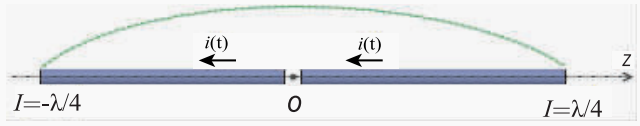长度为

l

l

的偶极子天线的电流分布可以表示为

I

(

z

)

=

I

0

sin

⁡

k

(

l

−

∣

z

∣

)

−

l

⩽

z

⩽

l

I(z)=I_{0} \sin k(l-|z|) \quad-l \leqslant z \leqslant l

式中，

I

0

I_{0}

是波腹电流;

k

k

是波数，且

k

=

2

π

/

λ

k=2π/λ

;

l

l

是偶极子天线的长度。对于半波偶极子天线而言，其长度

l

=

λ

/

4

l=λ/4

。把上述参数代入到上式中，则半波偶极子天线的电流分布可以改写为:

I

(

z

)

=

I

0

sin

⁡

(

π

2

−

k

z

)

=

I

0

cos

⁡

(

k

z

)

I(z)=I_{0} \sin \left(\frac{\pi}{2}-k z\right)=I_{0} \cos (k z)

2、辐射场和方向图
已知半波偶极子天线上的电流分布，可利用叠加原理来计算半波偶极子天线的辐射场。半波偶极子天线可以看成是由长度为dz的电基本振子天线连接而成的，dz 这一小段天线上的电流等幅同相，但沿着Z轴的电流幅度是按

I

(

z

)

=

I

0

cos

⁡

(

k

z

)

I(z)=I_{0} \cos (k z)

分布的。电基本振子的远区辐射场为

E

θ

=

j

I

d

l

2

λ

r

μ

0

ε

0

sin

⁡

θ

e

−

j

k

r

E_{\theta}=\mathrm{j} \frac{I d l}{2 \lambda r} \sqrt{\frac{\mu_{0}}{\varepsilon_{0}}} \sin \theta \mathrm{e}^{-\mathrm{j} k r}

对此进行积分得半波偶极子天线的辐射场为

E

θ

=

j

d

l

2

λ

r

μ

0

ε

0

sin

⁡

θ

e

−

j

k

r

[

∫

−

λ

/

4

λ

/

4

I

0

cos

⁡

(

k

z

)

d

z

]

E_{\theta}=\mathrm{j} \frac{d l}{2 \lambda r} \sqrt{\frac{\mu_{0}}{\varepsilon_{0}}} \sin \theta \mathrm{e}^{-\mathrm{jkr}}\left[\int_{-\lambda / 4}^{\lambda / 4} I_{0} \cos (k z) \mathrm{d} z\right]

整理可得

E

θ

=

j

60

I

0

r

cos

⁡

(

π

2

cos

⁡

θ

)

sin

⁡

θ

e

−

j

k

r

=

j

60

I

0

r

f

(

θ

,

φ

)

E_{\theta}=\mathrm{j} \frac{60 I_{0}}{r} \frac{\cos \left(\frac{\pi}{2} \cos \theta\right)}{\sin \theta} \mathrm{e}^{-\mathrm{j} k r}=\mathrm{j} \frac{60 I_{0}}{r} f(\theta, \varphi)

其中

f

(

θ

,

φ

)

=

f

(

θ

)

=

cos

⁡

(

π

2

cos

⁡

θ

)

sin

⁡

θ

f(\theta, \varphi)=f(\theta)=\frac{\cos \left(\frac{\pi}{2} \cos \theta\right)}{\sin \theta}

称为半波偶极子天线的方向性函数。 在电基本振子得辐射场中，电场分量

E

θ

E_{\theta}

和磁场分量

H

φ

H_{\varphi}

的比值为常数，将其称为波阻抗。对于自由空间而言，媒质的波阻抗为

η

0

=

E

θ

H

φ

=

μ

0

ε

0

=

120

π

Ω

\eta_{0}=\frac{E_{\theta}}{H_{\varphi}}=\sqrt{\frac{\mu_{0}}{\varepsilon_{0}}}=120 \pi \Omega

故可求得半波偶极子天线的磁场为

H

=

1

η

0

e

^

r

×

E

=

j

I

0

2

π

r

cos

⁡

(

π

2

cos

⁡

θ

)

sin

⁡

θ

e

−

j

k

r

e

^

φ

\boldsymbol{H}=\frac{1}{\eta_{0}} \widehat{\boldsymbol{e}}_{r} \times \boldsymbol{E}=\mathrm{j} \frac{I_{0}}{2 \pi r} \frac{\cos \left(\frac{\pi}{2} \cos \theta\right)}{\sin \theta} \mathrm{e}^{-\mathrm{j} k r} \widehat{\boldsymbol{e}}_{\varphi}

根据方向性函数可绘出半波偶极子天线的归一化场强方向图，在

H

H

平面（

θ

=

90

°

\theta=90°

）极坐标方向图是一个圆。在

E

E

平面（

φ

\varphi

为常数）中，辐射场强会随着角度

θ

\theta

的变化而变化，

θ

=

±

9

0

∘

\theta=\pm 90^{\circ}

方向上场强最大，

θ

=

0

∘

\theta=0^{\circ}

和

θ

=

18

0

∘

\theta=180^{\circ}

方向上场强为零。
3、方向性系数
天线的方向性系数

D

D

是指在远区场的某一球面上天线的辐射强度与平均辐射强度之比

D

(

θ

,

φ

)

=

U

(

θ

,

φ

)

U

0

D(\theta, \varphi)=\frac{U(\theta, \varphi)}{U_{0}}

式中，平均辐射强度

U

U

。实际上是辐射功率除以球面积，即:

U

0

=

1

4

π

∫

0

2

π

∫

0

π

U

(

θ

,

φ

)

sin

⁡

θ

d

θ

d

φ

U_{0}=\frac{1}{4 \pi} \int_{0}^{2 \pi} \int_{0}^{\pi} U(\theta, \varphi) \sin \theta \mathrm{d} \theta \mathrm{d} \varphi

通常所说的方向性系数指的都是在最大辐射方向上的方向性系数，即:

D

=

U

max

⁡

U

0

D=\frac{U_{\max }}{U_{0}}

代入方向性函数可计算出半波偶极子天线的功率方向性系数为

D

=

1

1

4

π

∫

0

2

π

∫

0

π

cos

⁡

2

θ

(

π

2

cos

⁡

θ

)

sin

⁡

2

θ

sin

⁡

θ

d

θ

d

φ

=

1.64

D=\frac{1}{\frac{1}{4 \pi} \int_{0}^{2 \pi} \int_{0}^{\pi} \frac{\cos ^{2} \theta\left(\frac{\pi}{2} \cos \theta\right)}{\sin ^{2} \theta} \sin \theta \mathrm{d} \theta \mathrm{d} \varphi}=1.64

即

D

d

B

=

10

lg

⁡

(

1.64

)

=

2.15

d

B

D_{\mathrm{dB}}=10 \lg (1.64)=2.15 \mathrm{~dB}

4、辐射电阻
天线的平均功率密度可以用平均坡印廷矢量来表示，即:

S

a

v

=

1

2

(

E

×

H

∗

)

\boldsymbol{S}_{a v}=\frac{1}{2}\left(\boldsymbol{E} \times \boldsymbol{H}^{*}\right)

把半波偶极子天线的辐射电场和辐射磁场代入式，可得

S

a

v

=

15

I

0

2

π

r

2

cos

⁡

2

(

π

2

cos

⁡

θ

)

sin

⁡

2

θ

\boldsymbol{S}_{a v}=\frac{15 I_{0}^{2}}{\pi r^{2}} \frac{\cos ^{2}\left(\frac{\pi}{2} \cos \theta\right)}{\sin ^{2} \theta}

半波偶极子天线的辐射功率则为:

S

r

=

∫

s

S

a

v

d

S

=

∫

0

2

π

∫

0

π

15

I

0

2

π

r

2

cos

⁡

2

θ

(

π

2

cos

⁡

θ

)

sin

⁡

2

θ

r

2

sin

⁡

θ

d

θ

d

φ

=

36.6

I

0

2

S_{r}=\int_{s} \boldsymbol{S}_{a v} \mathrm{~d} S=\int_{0}^{2 \pi} \int_{0}^{\pi} \frac{15 I_{0}^{2}}{\pi r^{2}} \frac{\cos ^{2} \theta\left(\frac{\pi}{2} \cos \theta\right)}{\sin ^{2} \theta} r^{2} \sin \theta \mathrm{d} \theta \mathrm{d} \varphi=36.6 I_{0}^{2}

这里使用R,来表示辐射电阻，有:

P

r

=

36.6

I

0

2

=

1

2

I

0

2

R

r

P_{r}=36.6 I_{0}^{2}=\frac{1}{2} I_{0}^{2} R_{r}

可以计算出半波偶极子天线的辐射电阻为:

R

r

=

73.2

Ω

R_{r}=73.2 \Omega

5、输入阻抗
根据基本的传输线理论，输入阻抗一般同时包含实部和虚部两部分，即为:

Z

i

n

=

R

i

n

+

j

X

i

n

Z_{\mathrm{in}}=R_{\mathrm{in}}+\mathrm{j} X_{\mathrm{in}}

其中，实部电阻

R

in

R_{\text{in}}

包含辐射电阻

R

r

R_{\text{r}}

,和导体损耗所产生的导体电阻

R

σ

R_{\sigma}

。对于良导体而言，导体电阻可以忽略，此时实部电阻仅包含辐射电阻，即为:

R

in

≈

R

r

R_{\text {in }} \approx R_{r}

由理论分析可知，偶极子天线在天线长度

2

l

2l

约为

λ

/

2

λ/2

时，虚部电抗

X

i

n

=

0

X_{\mathrm{in}}=0

。若采用更精确的场论分析，当

2

l

=

0.48

λ

2l=0.48λ

时，

X

i

n

=

0

X_{\mathrm{in}}=0

。综合以上的分析，对于半波偶极子天线而言，输入阻抗可以近似为:

Z

i

n

≈

R

r

=

73.2

Ω

Z_{\mathrm{in}} \approx R_{r}=73.2 \Omega

可见，半波偶极子天线的输入阻抗是纯电阻，易于和馈线匹配，这也是它被较多采用的原因之一。
展开全文射频工程
• 偶极天线： 两个导体之间有变化的电压 两种常见的天线都会产生意外的电磁波辐射，属于电子设备中隐藏的天线在辐射电磁波。 线路板上的闭合电路就构成了环形天线，产生的电磁波辐射属于差模辐射。 在"意外"回路中流动...
环形天线： 闭合回路中有变化的电流叫做环路天线。 偶极天线： 两个导体之间有变化的电压 两种常见的天线都会产生意外的电磁波辐射，属于电子设备中隐藏的天线在辐射电磁波。
线路板上的闭合电路就构成了环形天线，产生的电磁波辐射属于差模辐射。 在"意外"回路中流动的电流叫共模电流,共模电流的大小经常与”接地“的状态有关。 天线的互易性：一种结构如果有利于辐射电磁波，那就也有利于接收电磁波。
展开全文• 为解决因发射/接收天线相对位置变化而引起的信号衰减的问题，提出一种基于平面弯折偶极子结构的波束可控天线。该天线由6个独立的弯折偶极子辐射片与位于中心的反射片组成。通过改变馈源的位置，实现天线360°范围内......